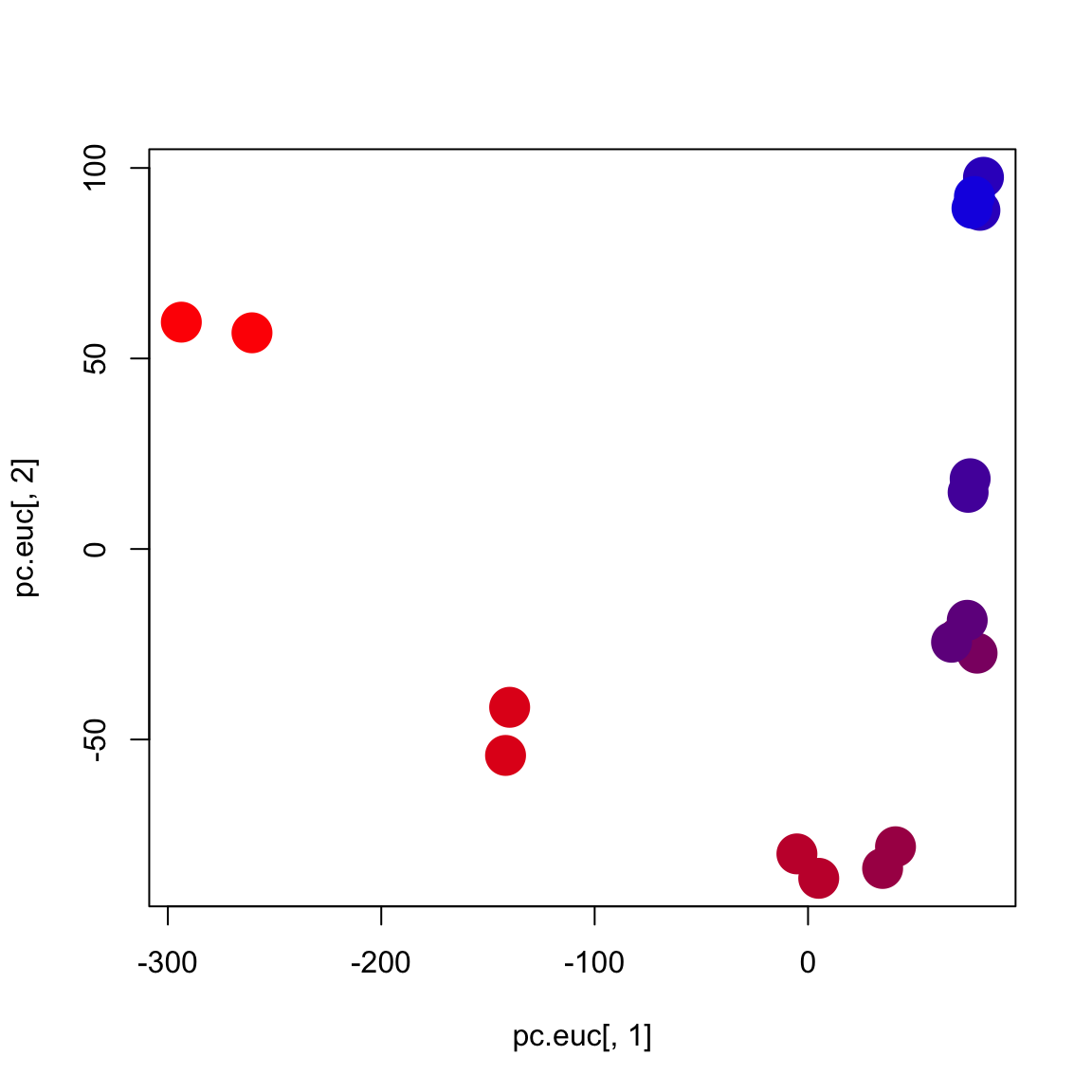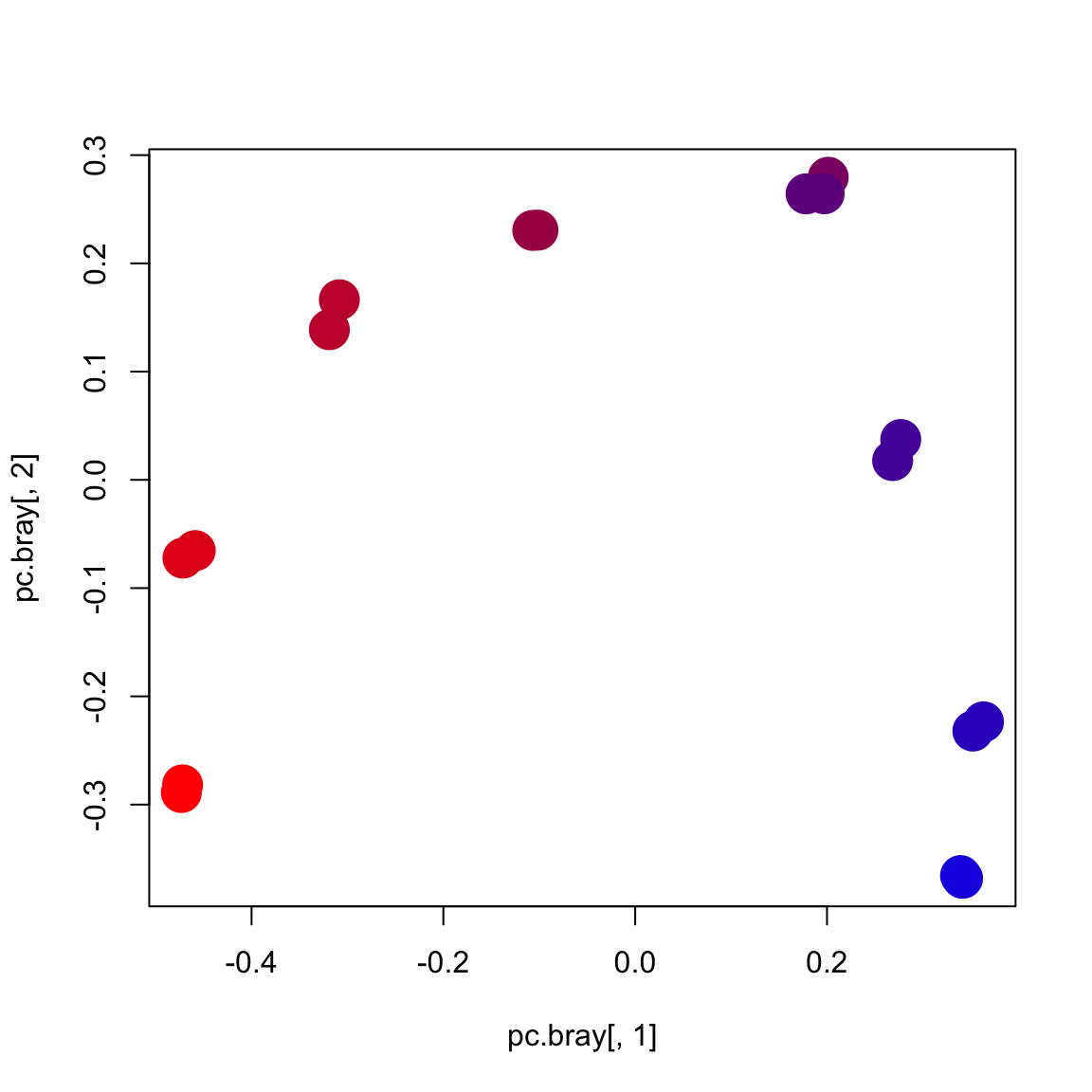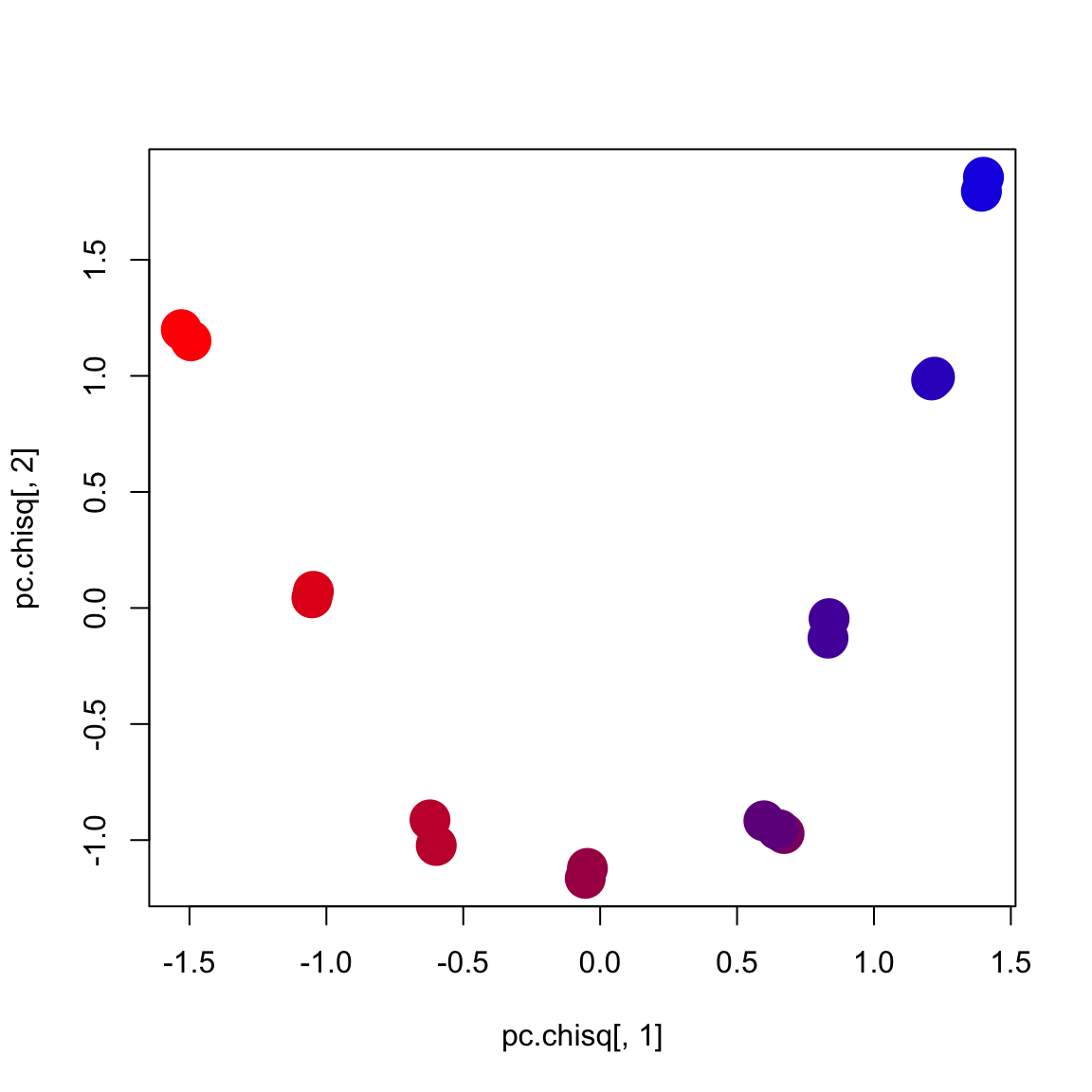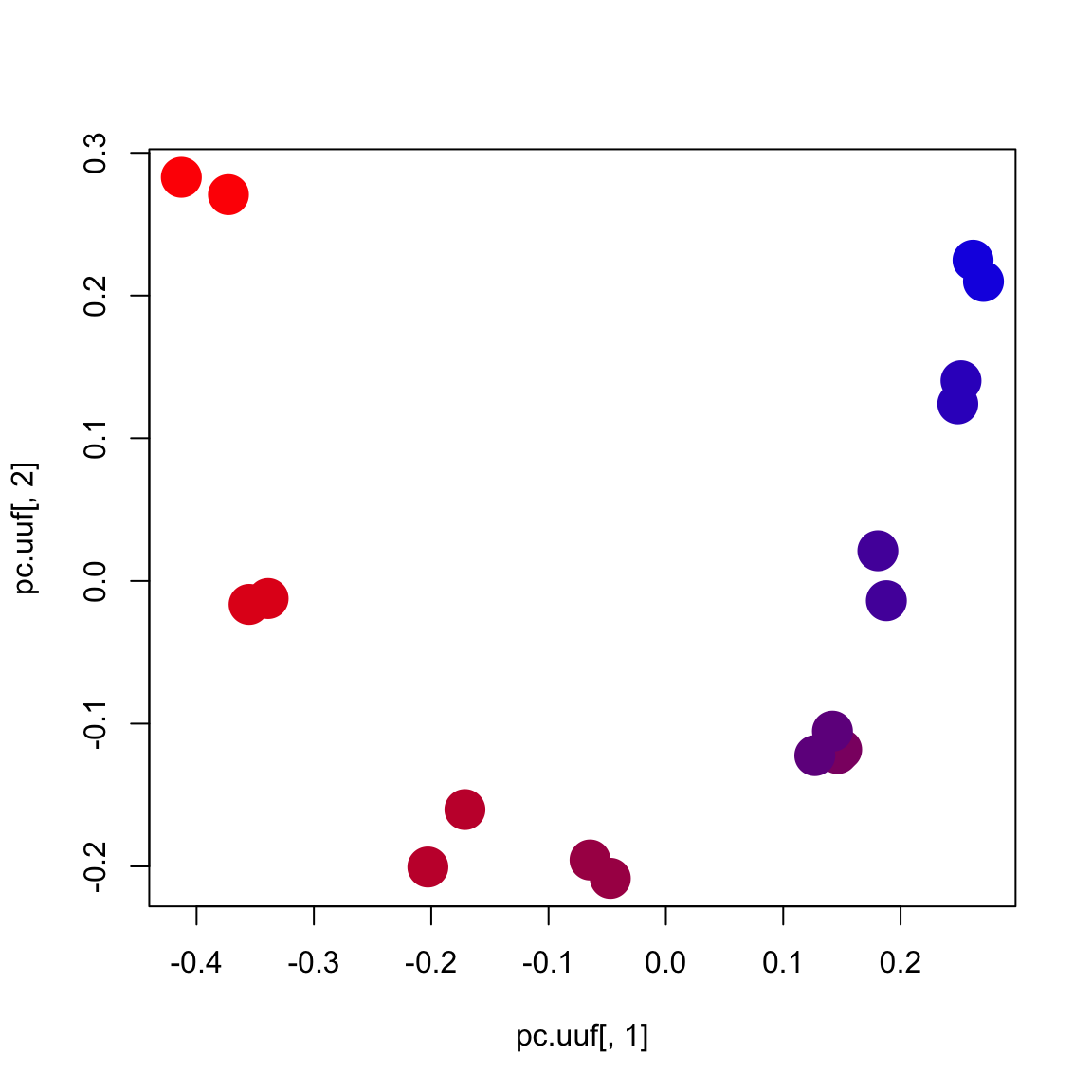All of the code in this page is meant to be run in `R` unless otherwise specified.

Install biom package and vegan package if not installed.

``install.packages(c('biom','vegan'),repo='http://cran.wustl.edu')``

``library('biom')``
``## Loading required package: methods``
``library('vegan')``
``## Warning: package 'vegan' was built under R version 3.2.3``
``## Loading required package: permute``
``## Warning: package 'permute' was built under R version 3.2.3``
``## Loading required package: lattice``
``## This is vegan 2.3-3``
``````# load biom file

# Extract data matrix (OTU counts) from biom table
otus <- as.matrix(biom_data(otus.biom))

# transpose so that rows are samples and columns are OTUs
otus <- t(otus)

It is extremely important to ensure that your OTU table and metadata table sample IDs are lined up correctly.

``````# see rownames of map and otus
rownames(map)``````
``````##   "GN01P.484257"   "GN01P.o.484256" "GN02P.o.484250" "GN02P.484248"
##   "GN03P.484253"   "GN03P.o.484249" "GN04P.484258"   "GN04P.o.484251"
##   "GN05P.o.484260" "GN05P.484261"   "GN06P.o.484262" "GN06P.484247"
##  "GN07P.o.484246" "GN07P.484259"   "GN08P.484265"   "GN08P.o.484263"
##  "GN09P.484254"   "GN09P.o.484264" "GN10P.o.484252" "GN10P.484255"``````
``rownames(otus)``
``````##   "GN01P.484257"   "GN07P.o.484246" "GN01P.o.484256" "GN06P.484247"
##   "GN05P.484261"   "GN08P.484265"   "GN05P.o.484260" "GN06P.o.484262"
##   "GN08P.o.484263" "GN07P.484259"   "GN04P.484258"   "GN04P.o.484251"
##  "GN09P.484254"   "GN09P.o.484264" "GN02P.484248"   "GN03P.484253"
##  "GN02P.o.484250" "GN03P.o.484249"``````
``````# find the overlap
common.ids <- intersect(rownames(map), rownames(otus))

# get just the overlapping samples
otus <- otus[common.ids,]
map <- map[common.ids,]``````

See dimensions of OTU table

``dim(otus)``
``##    18 1750``

See dimensions of mapping file

``dim(map)``
``##  18 60``

Get three different distances metrics

``````# get Euclidean distance
d.euc <- dist(otus)

# get Bray-Curtis distances (default for Vegan)
d.bray <- vegdist(otus)

# get Chi-square distances using vegan command
# we will extract chi-square distances from correspondence analysis
my.ca <- cca(otus)
d.chisq <- as.matrix(dist(my.ca\$CA\$u[,1:2]))``````

Now run principal coordinates embedding on the distance metrics

``````# Run PCoA (not PCA)
pc.euc <- cmdscale(d.euc, k=2)

# Bray-Curtis principal coords
pc.bray <- cmdscale(d.bray,k=2)

# get first two dimensions of chi-square coordinates:
pc.chisq <- my.ca\$CA\$u[,1:2]``````

Plot Euclidean distances with gradient colors

``````# makes a gradient from red to blue
my.colors <- colorRampPalette(c('red','blue'))(10)

# plot Euclidean PCoA coords using color gradient
# based on layer (1...10)
layer <- map[,'LAYER']
plot(pc.euc[,1], pc.euc[,2], col=my.colors[layer], cex=3, pch=16)``````Plot Bray-Curtis distances with gradient colors

``````# Plot Bray-Curtis PCoA
plot(pc.bray[,1], pc.bray[,2], col=my.colors[layer], cex=3, pch=16)``````Plot Chi-square distances with gradient colors

``````# Plot Chi-square PCoA
plot(pc.chisq[,1], pc.chisq[,2], col=my.colors[layer], cex=3, pch=16)``````## Visualizing UniFrac distances

Calculate UniFrac distances in QIIME

``````# Note: This command is on the command line, not in R
beta_diversity.py -i otu_table.biom -o beta -t ../ref/greengenes/97_otus.tree``````

``````# load unweighted and weighted unifrac

# ensure that these last two matrices have the same samples in the
# same order as the metadata table
d.uuf <- d.uuf[common.ids, common.ids]
d.wuf <- d.wuf[common.ids, common.ids]

# get first two dimensions of unifrac PCoA:
pc.uuf <- cmdscale(d.uuf, k=2)
pc.wuf <- cmdscale(d.wuf, k=2)``````

Plot unweighted UniFrac distances with gradient colors

``plot(pc.uuf[,1], pc.uuf[,2], col=my.colors[layer], cex=3, pch=16)``Plot weighted UniFrac distances with gradient colors

``plot(pc.wuf[,1], pc.wuf[,2], col=my.colors[layer], cex=3, pch=16)``